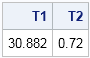In a previous post, I stated that you should avoid matrix multiplication that involves a huge diagonal matrix because that operation can be carried out more efficiently. Here's another tip that sometimes improves the efficiency of matrix multiplication: use parentheses to prevent the creation of large matrices.

Matrix multiplication is associative, which means that a matrix expression like Z = A*B*C can be computed as Z = (A*B)*C or as Z = A*(B*C). The answer is the same, but sometimes the dimensions of the matrices are such that one way is more efficient than the other. Because matrix languages such as the SAS/IML language evaluate matrix expressions from left to right, the expression Z = (A*B)*C is equivalent to not using parentheses at all.

When do parentheses help? Parentheses help when the product A*B is large, but B*C is small. The following SAS/IML statements compute the same product, but the computation that uses parentheses is much faster because it avoids forming a huge intermediate result:

```/* Compute Z = A*B*C where A is N x p matrix B is p x N matrix C is N x k matrix, and N is much large than p and k */ proc iml; N=10000; p = 500; k = 300; A = j(N,p,1); B = j(p,N,1); C = j(N,k,1);   t0 = time(); Z1 = A*B*C; T1 = time()-t0;   t0 = time(); Z2 = A*(B*C); T2 = time()-t0; print T1 T2;```This computation was done in SAS 9.4, which includes support for multithreaded matrix multiplication in SAS/IML. If you are running SAS 9.3, use a smaller value for N.

You can see that the first computation required about 30 seconds, whereas the second required less than a second. Both expressions compute the same N x k matrix. Why is the second computation so much faster?

• The first computation, must compute the temporary matrix A*B, which is an N x N matrix. After that huge matrix is formed, it is used to multiply C.
• The second computation computes the temporary matrix B*C, which is only a small p x k matrix. That matrix is premultiplied by the medium-sized matrix A to form the final result.

So in the second computation there is no "inflation" of the matrix sizes. Each intermediate matrix is the same size or smaller than the original matrices. In contrast, the first computation forms a huge matrix that is much larger than any of the original matrices.

So next time you need to compute a product of several matrices, think about the dimensions of those matrices and choose an order for the multiplication that will keep intermediate matrices small. The effect can be dramatic!

ShareDistinguished Researcher in Computational Statistics

Rick Wicklin, PhD, is a distinguished researcher in computational statistics at SAS and is a principal developer of SAS/IML software. His areas of expertise include computational statistics, simulation, statistical graphics, and modern methods in statistical data analysis. Rick is author of the books Statistical Programming with SAS/IML Software and Simulating Data with SAS.

1.Ian Wakeling on

That's really nice regarding the multithreading, in 9.4 my log reports a CPU time about 4 times greater than real time when I run the code above.

I am a bit confused by the quadratic form example, don't both ways involve the same amount of multiplication as x`*A is the same size as A*x?

•You are correct. In the original version of this post I had written the following:
"The most extreme example of this phenomenon is seen when computing a quadratic form such as y = x′*A*x, where x is a column vector and x′ is its transpose. It is always more efficient to compute a quadratic form as
y = x′*(A*x)"

As Ian points out, this statement is incorrect. I have no idea what I was thinking when I wrote it. Sorry for the confusion.

2.If your computer isn't too old there's no need to reduce N in case you are on SAS 9.3, I'd say. Just ran the code on my machine (i7 3.4GHz) and got
T1 T2
48.062 1.608

Thanks for this article Rick. Always good to be reminded of these things...